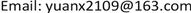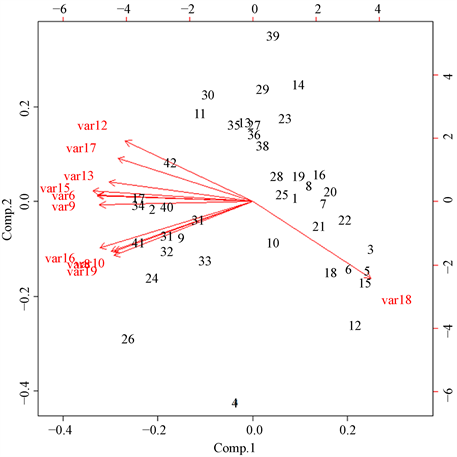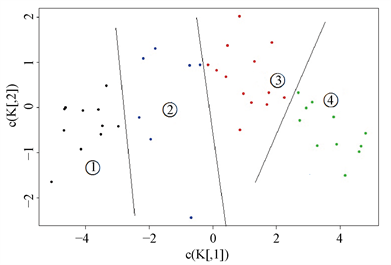﻿ 基于主成分分析的国家竞争力研究 Research on Competitiveness of Some Countries Based on Principal Component Analysis and K-Means Clustering

Vol. 08  No. 11 ( 2019 ), Article ID: 32974 , 9 pages
10.12677/ASS.2019.811255

Research on Competitiveness of Some Countries Based on Principal Component Analysis and K-Means Clustering

Zewei Zhang, Xiang Yuan

School of Economics & Management, Shanghai Maritime University, ShanghaiReceived: Oct. 25th, 2019; accepted: Nov. 8th, 2019; published: Nov. 15th, 2019ABSTRACT

In this paper, 20 evaluation indicators from 42 countries are selected. Firstly, the information entropy reflecting the degree of information disorder is used to preliminarily screen the indicators, and 12 indicators with high contribution rate are retained, thus achieving the effect of dimensionality reduction. Then the principal components representing most information are obtained by the principal components analysis and the results are clustered by K-means method. Finally, the ranking and classification of national competitiveness are obtained.

Keywords:Information Entropy, Principal Components Analysis, K-Means Clustering, National Competitiveness1. 引言

2. 研究方法

1) 对评价矩阵X消除量纲并做归一化处理后得到计算矩阵Y：

${y}_{ij}=\left({x}_{ij}-{\stackrel{¯}{x}}_{•j}\right)/\left(\mathrm{max}{x}_{•j}-\mathrm{min}{x}_{•j}\right)$

2) 然后计算每个指标的熵值，其中第j个指标的熵值为：

${H}_{j}=-k\underset{i=1}{\overset{n}{\sum }}{a}_{ij}\mathrm{ln}{a}_{ij}$ (式1)

3) 计算评价指标权重为：

${w}_{j}=\left(1-{H}_{j}\right)/\underset{j=1}{\overset{n}{\sum }}{H}_{j}$ (式2)

2.1. 指标体系构建Table 1. Index system of national competitiveness evaluation

2.2. 信息熵

2.3. 主成分分析

2.4. K-Means聚类

3. 实证分析

3.1. 数据来源

3.2. 利用信息熵对指标变量进行初步筛选

$\begin{array}{l}H=\left(2.574\text{\hspace{0.17em}}\text{\hspace{0.17em}}\text{\hspace{0.17em}}1.350\text{\hspace{0.17em}}\text{\hspace{0.17em}}\text{\hspace{0.17em}}2.784\text{\hspace{0.17em}}\text{\hspace{0.17em}}\text{\hspace{0.17em}}2.702\text{\hspace{0.17em}}\text{\hspace{0.17em}}\text{\hspace{0.17em}}3.370\text{\hspace{0.17em}}\text{\hspace{0.17em}}\text{\hspace{0.17em}}3.172\text{\hspace{0.17em}}\text{\hspace{0.17em}}\text{\hspace{0.17em}}2.813\text{\hspace{0.17em}}\text{\hspace{0.17em}}\text{\hspace{0.17em}}3.142\text{\hspace{0.17em}}\text{\hspace{0.17em}}\text{\hspace{0.17em}}3.546\text{\hspace{0.17em}}\text{\hspace{0.17em}}\text{\hspace{0.17em}}3.071\\ \text{\hspace{0.17em}}\text{\hspace{0.17em}}\text{\hspace{0.17em}}\text{\hspace{0.17em}}\text{\hspace{0.17em}}\text{\hspace{0.17em}}\text{ }\text{ }\text{\hspace{0.17em}}1.914\text{\hspace{0.17em}}\text{\hspace{0.17em}}\text{\hspace{0.17em}}3.448\text{\hspace{0.17em}}\text{\hspace{0.17em}}\text{\hspace{0.17em}}3.010\text{\hspace{0.17em}}\text{\hspace{0.17em}}\text{\hspace{0.17em}}2.702\text{\hspace{0.17em}}\text{\hspace{0.17em}}\text{\hspace{0.17em}}3.366\text{\hspace{0.17em}}\text{\hspace{0.17em}}\text{\hspace{0.17em}}3.495\text{\hspace{0.17em}}\text{\hspace{0.17em}}\text{\hspace{0.17em}}3.250\text{\hspace{0.17em}}\text{\hspace{0.17em}}\text{\hspace{0.17em}}3.132\text{\hspace{0.17em}}\text{\hspace{0.17em}}\text{\hspace{0.17em}}3.308\text{\hspace{0.17em}}\text{\hspace{0.17em}}\text{\hspace{0.17em}}2.478\right)\end{array}$

$\begin{array}{l}W=\left(0.041\text{\hspace{0.17em}}\text{\hspace{0.17em}}\text{\hspace{0.17em}}0.009\text{\hspace{0.17em}}\text{\hspace{0.17em}}\text{\hspace{0.17em}}0.046\text{\hspace{0.17em}}\text{\hspace{0.17em}}\text{\hspace{0.17em}}0.044\text{\hspace{0.17em}}\text{\hspace{0.17em}}\text{\hspace{0.17em}}0.061\text{\hspace{0.17em}}\text{\hspace{0.17em}}\text{\hspace{0.17em}}0.056\text{\hspace{0.17em}}\text{\hspace{0.17em}}\text{\hspace{0.17em}}0.047\text{\hspace{0.17em}}\text{\hspace{0.17em}}\text{\hspace{0.17em}}0.055\text{\hspace{0.17em}}\text{\hspace{0.17em}}\text{\hspace{0.17em}}0.066\text{\hspace{0.17em}}\text{\hspace{0.17em}}\text{\hspace{0.17em}}0.054\\ \text{\hspace{0.17em}}\text{\hspace{0.17em}}\text{\hspace{0.17em}}\text{\hspace{0.17em}}\text{\hspace{0.17em}}\text{\hspace{0.17em}}\text{ }\text{ }\text{\hspace{0.17em}}0.024\text{\hspace{0.17em}}\text{\hspace{0.17em}}\text{\hspace{0.17em}}0.063\text{\hspace{0.17em}}\text{\hspace{0.17em}}\text{\hspace{0.17em}}0.052\text{\hspace{0.17em}}\text{\hspace{0.17em}}\text{\hspace{0.17em}}0.044\text{\hspace{0.17em}}\text{\hspace{0.17em}}\text{\hspace{0.17em}}0.061\text{\hspace{0.17em}}\text{\hspace{0.17em}}\text{\hspace{0.17em}}0.065\text{\hspace{0.17em}}\text{\hspace{0.17em}}\text{\hspace{0.17em}}0.058\text{\hspace{0.17em}}\text{\hspace{0.17em}}\text{\hspace{0.17em}}0.055\text{\hspace{0.17em}}\text{\hspace{0.17em}}\text{\hspace{0.17em}}0.060\text{\hspace{0.17em}}\text{\hspace{0.17em}}\text{\hspace{0.17em}}0.038\right)\end{array}$

3.3. 利用主成分分析对指标变量进行降维

3.3.1. 求出12个变量的相关系数矩阵，特征值和特征向量

3.3.2. 主成分分析

$\begin{array}{c}{y}_{1}=-0.311{\mathrm{var}}_{5}^{\ast }-0.312{\mathrm{var}}_{6}^{\ast }-0.284{\mathrm{var}}_{8}^{\ast }-0.308{\mathrm{var}}_{9}^{\ast }-0.278{\mathrm{var}}_{10}^{\ast }-0.256{\mathrm{var}}_{12}^{\ast }\\ \text{\hspace{0.17em}}\text{\hspace{0.17em}}-0.288{\mathrm{var}}_{13}^{\ast }-0.321{\mathrm{var}}_{15}^{\ast }-0.307{\mathrm{var}}_{16}^{\ast }-0.271{\mathrm{var}}_{17}^{\ast }+0.237{\mathrm{var}}_{18}^{\ast }-0.279{\mathrm{var}}_{19}^{\ast }\end{array}$

$\begin{array}{c}{y}_{2}=-0.335{\mathrm{var}}_{8}^{\ast }-0.335{\mathrm{var}}_{10}^{\ast }+0.409{\mathrm{var}}_{12}^{\ast }+0.132{\mathrm{var}}_{13}^{\ast }\\ \text{\hspace{0.17em}}\text{\hspace{0.17em}}-0.311{\mathrm{var}}_{16}^{\ast }+0.292{\mathrm{var}}_{17}^{\ast }-0.517{\mathrm{var}}_{18}^{\ast }-0.364{\mathrm{var}}_{19}^{\ast }\end{array}$

$\begin{array}{c}{y}_{3}=0.138{\mathrm{var}}_{6}^{\ast }-0.284{\mathrm{var}}_{9}^{\ast }+0.111{\mathrm{var}}_{10}^{\ast }+0.583{\mathrm{var}}_{12}^{\ast }\\ \text{\hspace{0.17em}}\text{\hspace{0.17em}}-0.402{\mathrm{var}}_{13}^{\ast }+0.376{\mathrm{var}}_{17}^{\ast }+0.483{\mathrm{var}}_{19}^{\ast }\end{array}$

3.3.3. 主成分得分Table 4. Scoring and ranking of national competitiveness evaluationFigure 1. Double projection

3.3.4. K-Means聚类Figure 2. K-means clustering scatter

4. 对实例的相关思考

Research on Competitiveness of Some Countries Based on Principal Component Analysis and K-Means Clustering[J]. 社会科学前沿, 2019, 08(11): 1873-1881. https://doi.org/10.12677/ASS.2019.811255

1. 1. 迈克尔•波特. 国家竞争优势[M]. 北京: 华夏出版社, 2002.

2. 2. Van Wyk, J. (2010) Double Diamonds, Real Diamonds: Botswana’s National Competitiveness Academy of Marketing Studies Journal, 14, 55-76.

3. 3. 易顺, 韩江波. 国外国家竞争力研究: 理论架构与展望[J]. 兰州商学院学报, 2014(5): 93-102.

4. 4. 魏海燕.《世界竞争力年鉴》评价体系研究及其思考[J]. 科技管理研究, 2013(5): 65-68+77.

5. 5. 田美玲, 方世明. 汉江流域中心城市竞争力的评价及时空演变[J]. 统计与决策, 2016(9): 103-106.

6. 6. 于丽英, 郭洪晶. 长江三角洲地区城市综合竞争力的评价研究[J]. 上海大学学报(社会科学版), 2011, 18(1): 79-90.